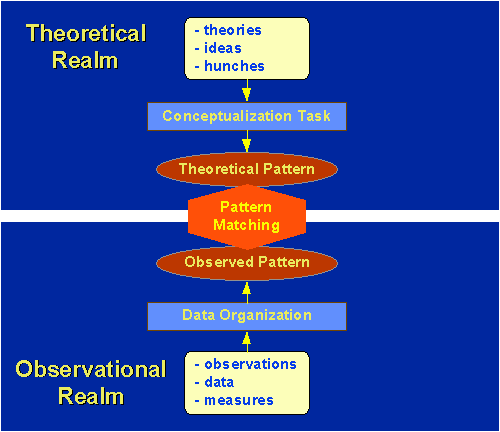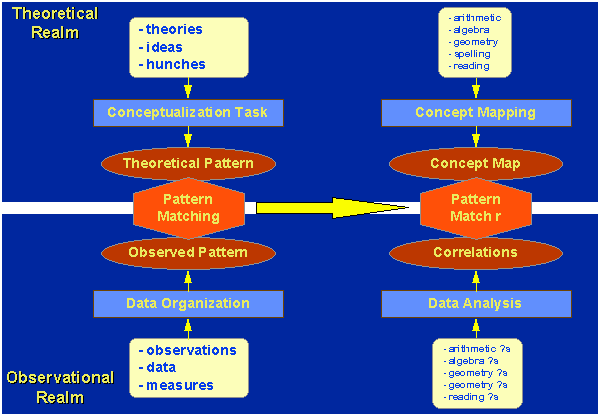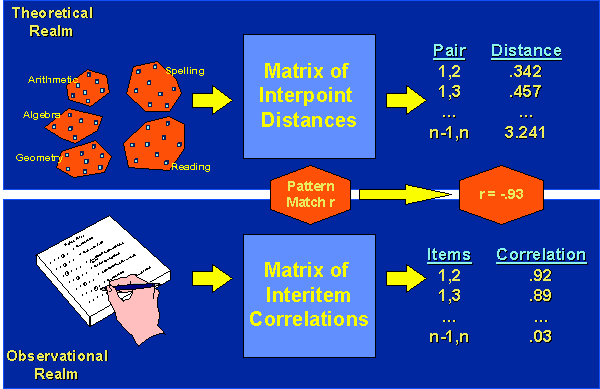Pattern Matching

# Pattern Matching for Construct Validity

The idea of using pattern matching as a rubric for assessing construct validity is an area where I have tried to make a contribution (Trochim, W., (1985). Pattern matching, validity, and conceptualization in program evaluation. Evaluation Review, 9, 5, 575-604 and Trochim, W. (1989). Outcome pattern matching and program theory. Evaluation and Program Planning, 12, 355-366.), although my work was very clearly foreshadowed, especially in much of Donald T. Campbell’s writings. Here, I’ll try to explain what I mean by pattern matching with respect to construct validity.

## The Theory of Pattern Matching

A pattern is any arrangement of objects or entities. The term “arrangement” is used here to indicate that a pattern is by definition non-random and at least potentially describable. All theories imply some pattern, but theories and patterns are not the same thing. In general, a theory postulates structural relationships between key constructs. The theory can be used as the basis for generating patterns of predictions. For instance, E=MC2 can be considered a theoretical formulation. A pattern of expectations can be developed from this formula by generating predicted values for one of these variables given fixed values of the others. Not all theories are stated in mathematical form, especially in applied social research, but all theories provide information that enables the generation of patterns of predictions.Pattern matching always involves an attempt to link two patterns where one is a theoretical pattern and the other is an observed or operational one. The top part of the figure shows the realm of theory. The theory might originate from a formal tradition of theorizing, might be the ideas or “hunches” of the investigator, or might arise from some combination of these. The conceptualization task involves the translation of these ideas into a specifiable theoretical pattern indicated by the top shape in the figure. The bottom part of the figure indicates the realm of observation. This is broadly meant to include direct observation in the form of impressions, field notes, and the like, as well as more formal objective measures. The collection or organization of relevant operationalizations (i.e., relevant to the theoretical pattern) is termed the observational pattern and is indicated by the lower shape in the figure. The inferential task involves the attempt to relate, link or match these two patterns as indicated by the double arrow in the center of the figure. To the extent that the patterns match, one can conclude that the theory and any other theories which might predict the same observed pattern receive support.

It is important to demonstrate that there are no plausible alternative theories that account for the observed pattern and this task is made much easier when the theoretical pattern of interest is a unique one. In effect, a more complex theoretical pattern is like a unique fingerprint which one is seeking in the observed pattern. With more complex theoretical patterns it is usually more difficult to construe sensible alternative patterns that would also predict the same result. To the extent that theoretical and observed patterns do not match, the theory may be incorrect or poorly formulated, the observations may be inappropriate or inaccurate, or some combination of both states may exist.

All research employs pattern matching principles, although this is seldom done consciously. In the traditional two-group experimental context, for instance, the typical theoretical outcome pattern is the hypothesis that there will be a significant difference between treated and untreated groups. The observed outcome pattern might consist of the averages for the two groups on one or more measures. The pattern match is accomplished by a test of significance such as the t-test or ANOVA. In survey research, pattern matching forms the basis of generalizations across different concepts or population subgroups. In qualitative research pattern matching lies at the heart of any attempt to conduct thematic analyses.

While current research methods can be described in pattern matching terms, the idea of pattern matching implies more, and suggests how one might improve on these current methods. Specifically, pattern matching implies that more complex patterns, if matched, yield greater validity for the theory. Pattern matching does not differ fundamentally from traditional hypothesis testing and model building approaches. A theoretical pattern is a hypothesis about what is expected in the data. The observed pattern consists of the data that are used to examine the theoretical model. The major differences between pattern matching and more traditional hypothesis testing approaches are that pattern matching encourages the use of more complex or detailed hypotheses and treats the observations from a multivariate rather than a univariate perspective.

## Pattern Matching and Construct Validity

While pattern matching can be used to address a variety of questions in social research, the emphasis here is on its use in assessing construct validity.The accompanying figure shows the pattern matching structure for an example involving five measurement constructs – arithmetic, algebra, geometry, spelling, and reading. In this example, we’ll use concept mapping to develop the theoretical pattern among these constructs. In the concept mapping we generate a large set of potential arithmetic, algebra, geometry, spelling, and reading questions. We sort them into piles of similar questions and develop a map that shows each question in relation to the others. On the map, questions that are more similar are closer to each other, those less similar are more distant. From the map, we can find the straight-line distances between all pair of points (i.e., all questions). This is the matrix of interpoint distances. We might use the questions from the map in constructing our measurement instrument, or we might sample from these questions. On the observed side, we have one or more test instruments that contain a number of questions about arithmetic, algebra, geometry, spelling, and reading. We analyze the data and construct a matrix of inter-item correlations.

What we want to do is compare the matrix of interpoint distances from our concept map (i.e., the theoretical pattern) with the correlation matrix of the questions (i.e., the observed pattern). How do we achieve this? Let’s assume that we had 100 prospective questions on our concept map, 20 for each construct. Correspondingly, we have 100 questions on our measurement instrument, 20 in each area. Thus, both matrices are 100x100 in size. Because both matrices are symmetric, we actually have (N(N-1))/2 = (100(99))/2 = 99002 = 4,950 unique pairs (excluding the diagonal). If we “string out” the values in each matrix we can construct a vector or column of 4,950 numbers for each matrix. The first number is the value comparing pair (1,2), the next is (1,3) and so on to (N-1, N) or (99, 100). Now, we can compute the overall correlation between these two columns, which is the correlation between our theoretical and observed patterns, the “pattern matching correlation.” In this example, let’s assume it is -.93. Why would it be a negative correlation? Because we are correlating distances on the map with the similarities in the correlations and we expect that greater distance on the map should be associated with lower correlation and less distance with greater correlation.

The pattern matching correlation is our overall estimate of the degree of construct validity in this example because it estimates the degree to which the operational measures reflect our theoretical expectations.